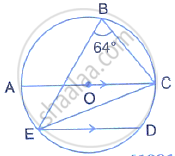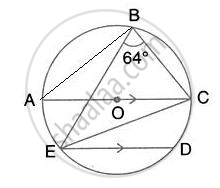Share

# In the Given Figure, Aoc is a Diameter and Ac is Parallel to Ed. If ∠Cbe = 64°, Calculate ∠Dec . - Mathematics

Course

#### Question

In the given figure, AOC is a diameter and AC is parallel to ED. If ∠CBE = 64°, Calculate ∠DEC .#### SolutionJoin AB,
∠ABC = 90°
(Angle in a semi circle)
∴ ∠ABE = 90° - 64° = 26°

Now, ∠ABE = ∠ACE = 26°

(Angle in the same segment)

Also, AC || ED
∴ ∠DEC = ∠ACE = 26°     (Alternate angles)

Is there an error in this question or solution?